# Add and Subtract Mixed Number Fractions

In this worksheet, students will add and subtract mixed number fractions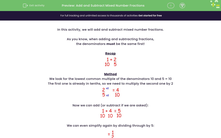Key stage:  KS 3

Curriculum topic:   Number

Curriculum subtopic:   Use Four Operations for All Numbers

Difficulty level:#### Worksheet Overview

In this activity, we will add and subtract mixed number fractions.

As you know, when adding and subtracting fractions,

the denominators must be the same first!

Recap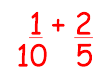Method

We look for the lowest common multiple of the denominators 10 and 5 = 10

The first one is already in tenths, so we need to multiply the second one by 2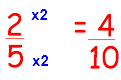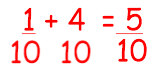We can even simplify again by dividing through by 5: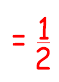In this activity, we will look at adding with mixed number fractions.

Example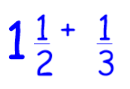Step 1

Convert any mixed number fractions to improper fractions: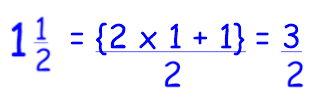Step 2

Rewrite the question with improper fractions: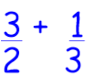Step 3

We look for the lowest common multiple of the denominators 2 and 3 = 6

Multiply fractions to make them equivalent: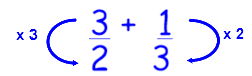Step 4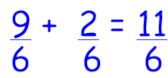Step 5

Convert back to a mixed number:

# 11 ÷ 6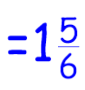This is quite a long process but you will get used to it!

In the meantime, follow the steps!Let's have a go at some questions now.

### What is EdPlace?

We're your National Curriculum aligned online education content provider helping each child succeed in English, maths and science from year 1 to GCSE. With an EdPlace account you’ll be able to track and measure progress, helping each child achieve their best. We build confidence and attainment by personalising each child’s learning at a level that suits them.

Get started